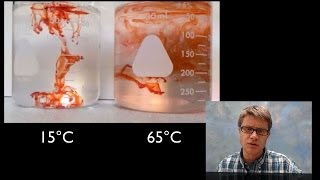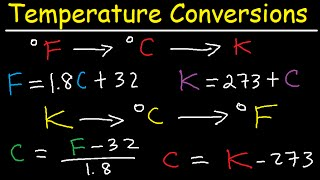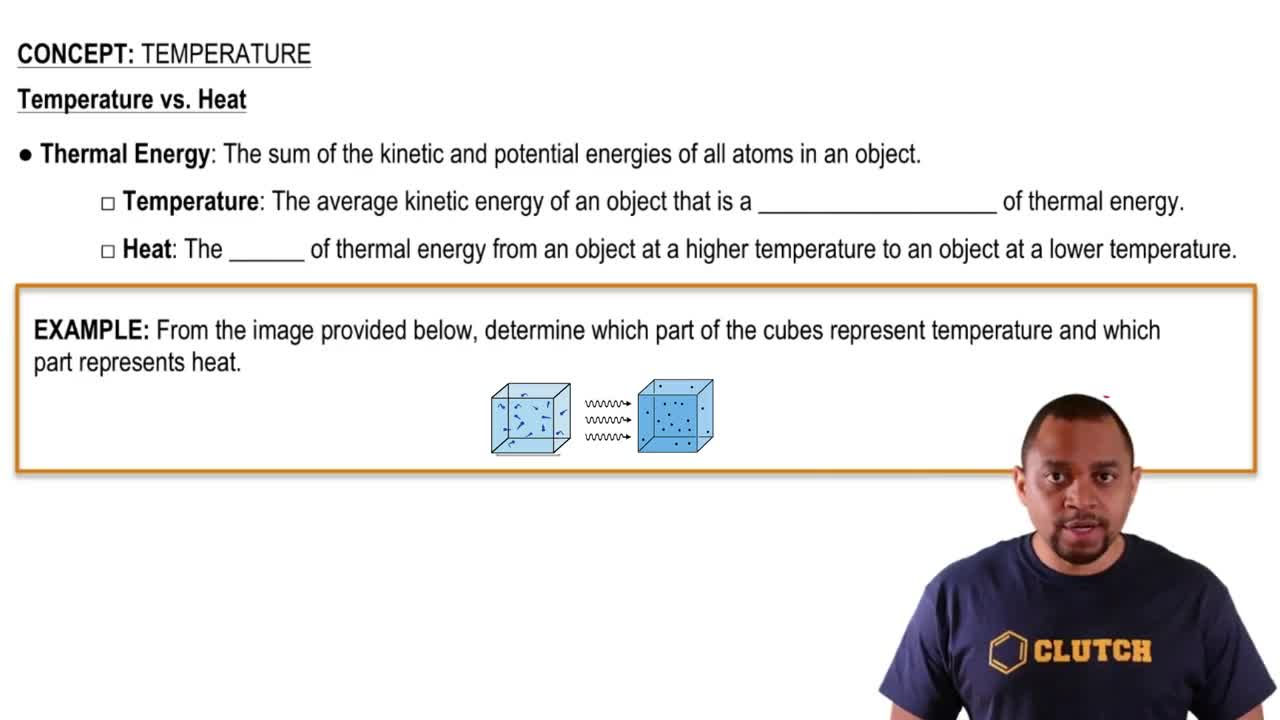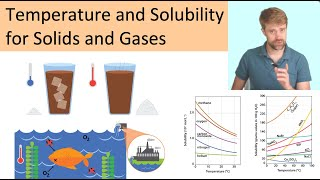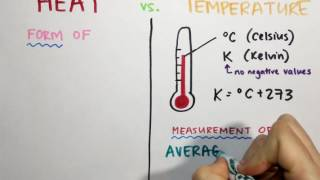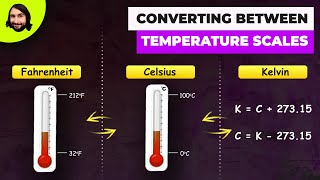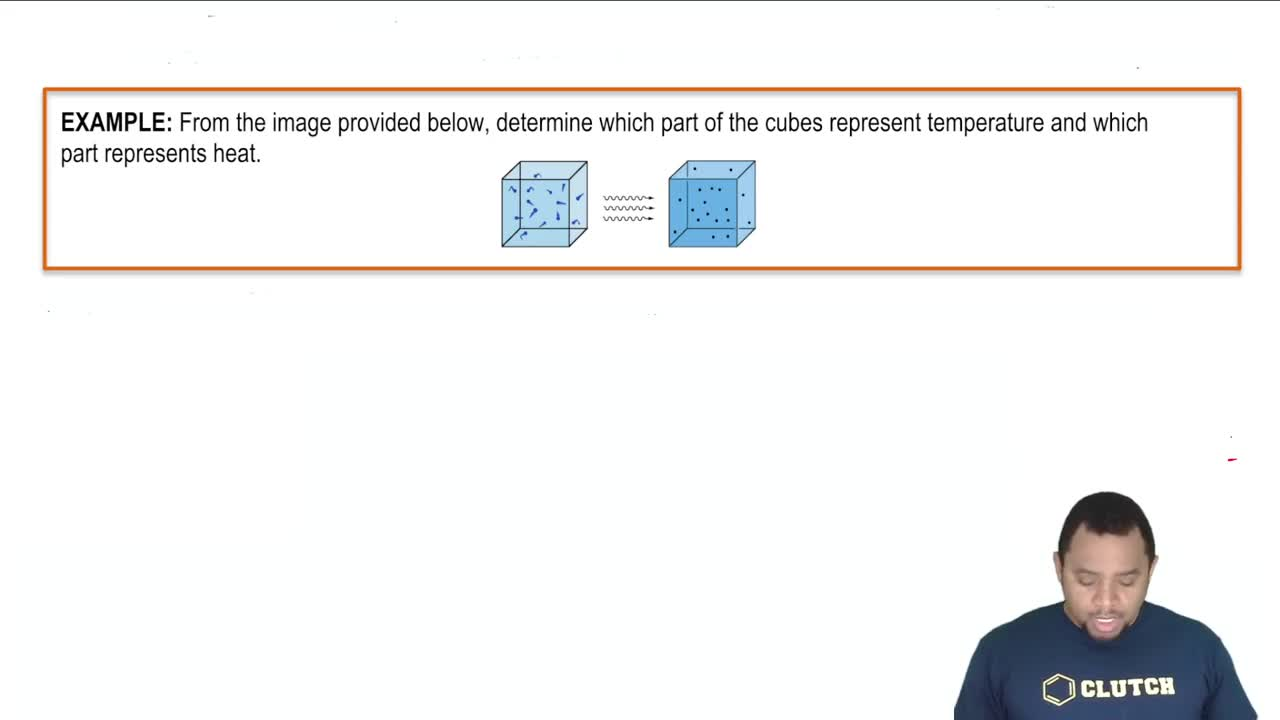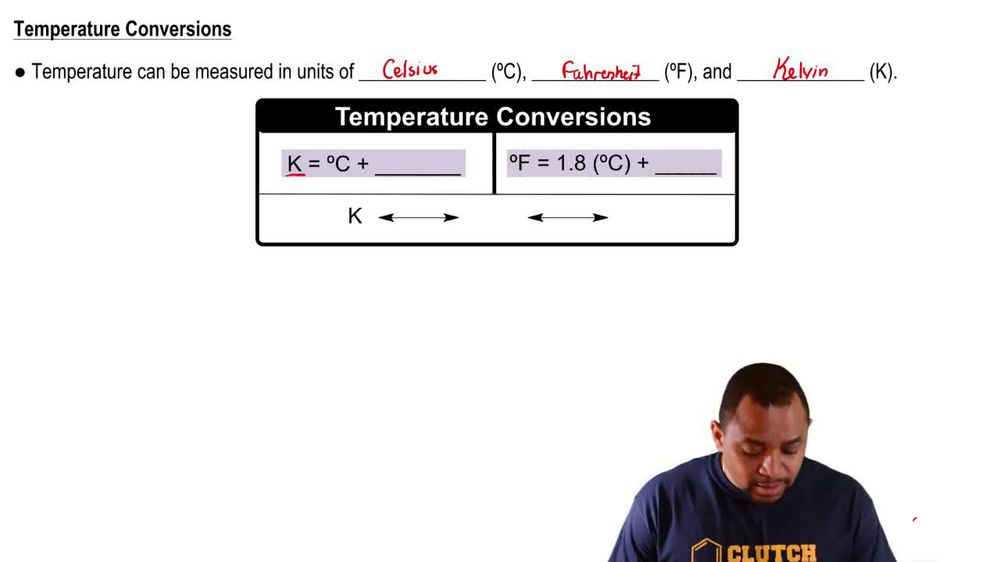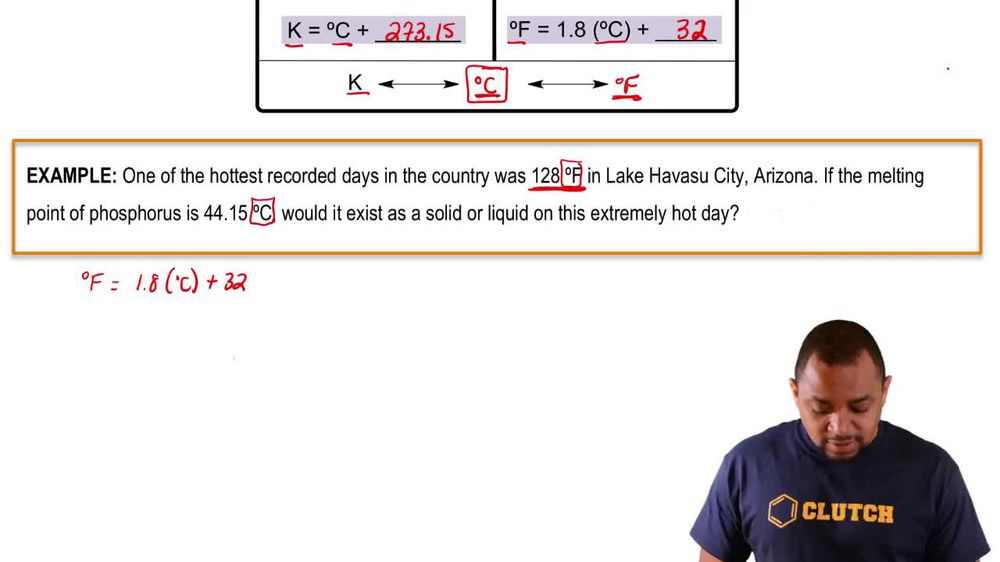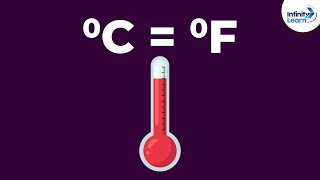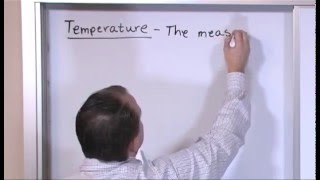Start typing, then use the up and down arrows to select an option from the list.
1. 1. Intro to General Chemistry2. Temperature
Problem

# The Rankine temperature scale used in engineering is to the Fahrenheit scale as the Kelvin scale is to the Celsius scale. That is, 1 Rankine degree is the same size as 1 Fahrenheit degree, and 0 °R = absolute zero. (a) What temperature corresponds to the freezing point of water on the Rankine scale?

Relevant Solution1m
Play a video:
Hello everyone today. What you're being given the falling problem? The newton scale was created by Isaac Newton for practical uses. Where he said zero as the freezing point of water. What temperature on the newton scale corresponds to the freezing point of methanol? So on the Celsius scale, So on the Celsius scale, methanol Equals -97.6°C. And so if we take our formula here, we'll take our degrees and newton will equal out to our degrees Celsius. But multiplying that by R33 over We get negative 97.6°C times are 33 over 100. This gives us - .208° Newton as our final answer. Or, better yet, For better significant figures, this would just be negative 32.2° Newton. And with that we've answered the question overall. I hope this helped. And until next time.